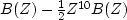Next: Convolution with Z-transform Up: SAMPLED DATA AND Z-TRANSFORMS Previous: SAMPLED DATA AND Z-TRANSFORMS

## Linear superposition

If the first explosion were followed by an implosion of half-strength, we would have.If pulses overlapped one another in time (as would be the case if B(Z) had degree greater than 10), the waveforms would simply add together in the region of overlap. The supposition that they would just add together without any interaction is called the linearity'' property. In seismology we find that--although the earth is a heterogeneous conglomeration of rocks of different shapes and types--when seismic waves travel through the earth, they do not interfere with one another. They satisfy linear superposition. The plague of nonlinearity arises from large amplitude disturbances. Nonlinearity is a dominating feature in hydrodynamics, where flow velocities are a noticeable fraction of the wave velocity. Nonlinearity is absent from reflection seismology except within a few meters from the source. Nonlinearity does not arise from geometrical complications in the propagation path. An example of two plane waves superposing is shown in Figure 4.

 super Figure 4 Crossing plane waves superposing viewed on the left as wiggle traces'' and on the right as raster.''Next: Convolution with Z-transform Up: SAMPLED DATA AND Z-TRANSFORMS Previous: SAMPLED DATA AND Z-TRANSFORMS
Stanford Exploration Project
10/21/1998